#### How to get rid of circular references?

In this Excel tutorial, you will learn how to remove the circular references in an Excel file.

## What is a circular reference?

Let us understand what circular reference is. A circular reference happens when a formula directly or indirectly refers to its own cell. This causes the formula to use the result in the calculation and it runs in a loop, causing an error. When there are circular reference errors, Excel cannot do the other formula calculations automatically.

If you leave them in, Excel calculates each cell involved in the circular reference by using the results of the previous iteration. An iteration is a repeated recalculation until a specific numerical condition is met. By default, Excel stops calculating after 100 iterations or after all values in the circular reference change by less than 0.001 between iterations, unless you change the Excel option.

A circular reference can cause an infinite loop in the spreadsheet.

Let’s create a circular reference now to look at it:

I wrote a value in cell A3, which is 10.

In cell B3 I wrote a formula: = A3+B3.

We are already putting a formula in B3 and using the same in the formula.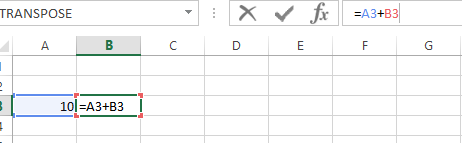On clicking enter/tab, it gives the following error:On clicking ok it gives the wrong results = 0 and at the bottom it keeps showing a warning: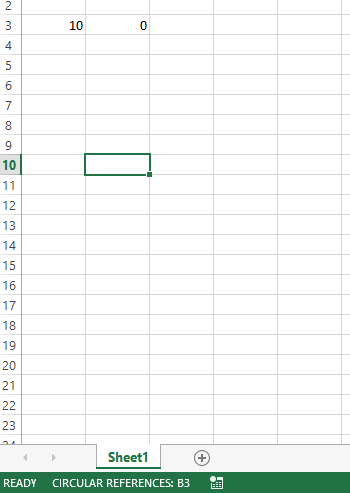## How to find the circular reference?

Circular references occur in Excel when a formula refers to itself either directly or indirectly. This can lead to incorrect or unpredictable results, and it’s important to resolve them.

Here are some steps to help you get rid of circular references in Excel:

1. Identify the cells that are causing the circular reference. Excel will usually display a warning message when a circular reference is detected, which can help you identify the cells involved.
2. Review the formulas in the cells identified in step 1 to determine if they are causing the circular reference. Check if any of the cells are referencing themselves or if there are any circular references involving other cells.
3. Correct the formula(s) causing the circular reference. You can do this by editing the formula(s) in the affected cells to remove the reference to itself or to break the circular reference chain.
4. If you’re having trouble identifying the cells causing the circular reference, you can use Excel’s Trace Dependents and Trace Precedents features to help you. These features will show you which cells are dependent on or precede the selected cell.
5. If you need to use circular references in your Excel model, you can enable iterative calculations in Excel. This feature allows Excel to calculate a formula multiple times until it reaches a specified result or limit.

Once you’ve corrected the circular reference, save your workbook to ensure that the changes are retained.

By following these steps, you can effectively get rid of circular references in Excel and ensure that your formulas are working correctly.

1. Go to the Formulas tab.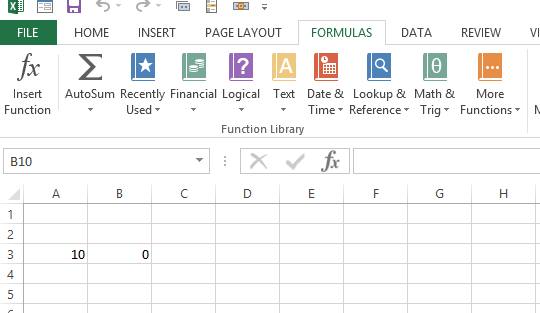2.Click on error checking: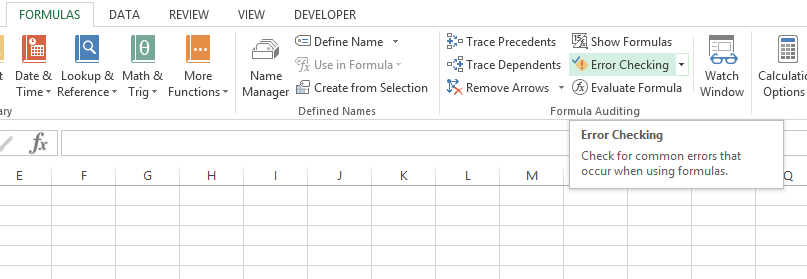In error checking, click on circular references: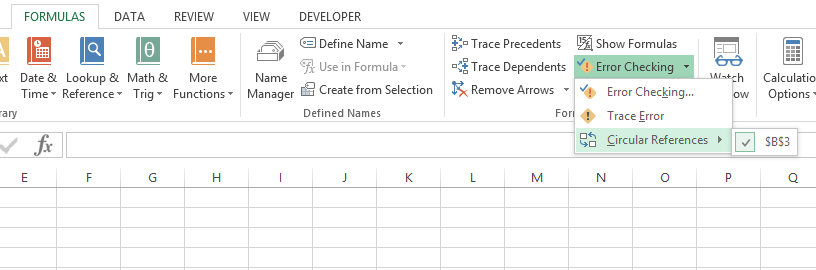It shows the cells that have the issue, and we can correct them.

If you cannot figure out if the cell is the cause of the circular reference, click the next cell in the Circular References submenu, if available.

Continue to review and correct the circular reference until the status bar no longer displays the word “Circular”.

Now we will learn how to remove the circular references.

## How to enable / disable circular references in Excel

As noted earlier, iterative calculations are usually turned off in Excel by default (in this context, iteration is the repeated recalculation until a specific numeric condition is met). For circular formulas to work, you must enable iterative calculations in your Excel workbook.

Click File > Options, go to Formulas, and select the Enable iterative calculation check box under the Calculation options section.

When you turn on iterative calculations, you must specify the following two options:

1. Maximum Iterations box – specifies how many times the formula should be recalculated. The higher the number of iterations, the more time the calculation takes.
2. Maximum Change box – specifies the maximum change between calculation results. The smaller the number, the more accurate the result you get and the more time Excel takes to calculate the worksheet. The default settings are 100 for Maximum Iterations, and 0.001 for Maximum Change. What it means is that Microsoft Excel will stop calculating your circular formula after 100 iterations or after a change of less than 0.001 between iterations, whichever comes first.